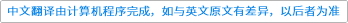Applied Mathematics and Computation ( IF 3.472 ) Pub Date : 2021-01-13 , DOI: 10.1016/j.amc.2020.125872
Yusheng Zhou; Danhong Chen

This paper proposes a new method for designing a state-dependent switching law to stabilize a class of switched nonlinear system with two unstable subsystems. The main idea is to convert each subsystem to a second-order mechanical system by introducing a reversible transformation, thus the summation of its kinetic and potential energies is calculated as an energy function. Then, by defining a performance index with energy function and using the variational principle, two optimal switching curves are derived from the Euler equation. By adopting a switching law designed by such switching curves, the state of the switched system can approach to the origin at the fastest speed in a sense. In addition, for the case of switched systems with linear vibration subsystems, a critical stability condition related to the stiffness and negative damping coefficients of the subsystems is obtained to make the switched system periodic. Finally, simulation results show that the proposed method can effectively solve the stability problem of switched systems, in which each subsystem does not have any stability factors.down
wechat
bug